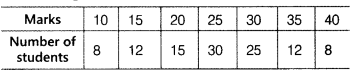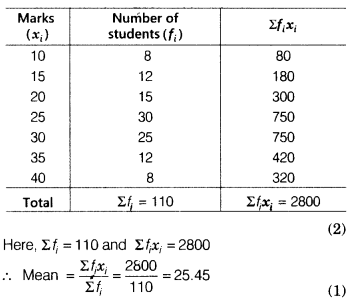# Calculate the mean and mode for the following data

Calculate the mean and mode for the following data.The table for marks and number of students is given belowWe know that mode is the value, which contain highest frequency.
Here, we see in the given table that 30 is the highest frequency and its corresponding marks is 25.
Hence, mode of the given data is 25.